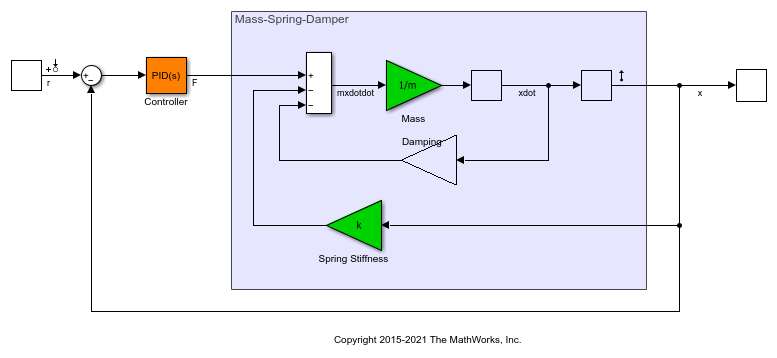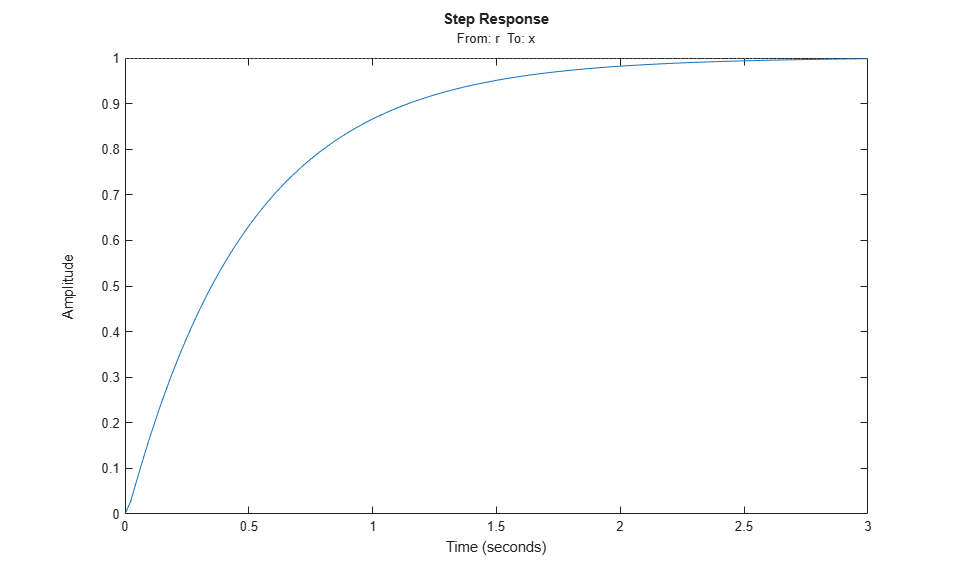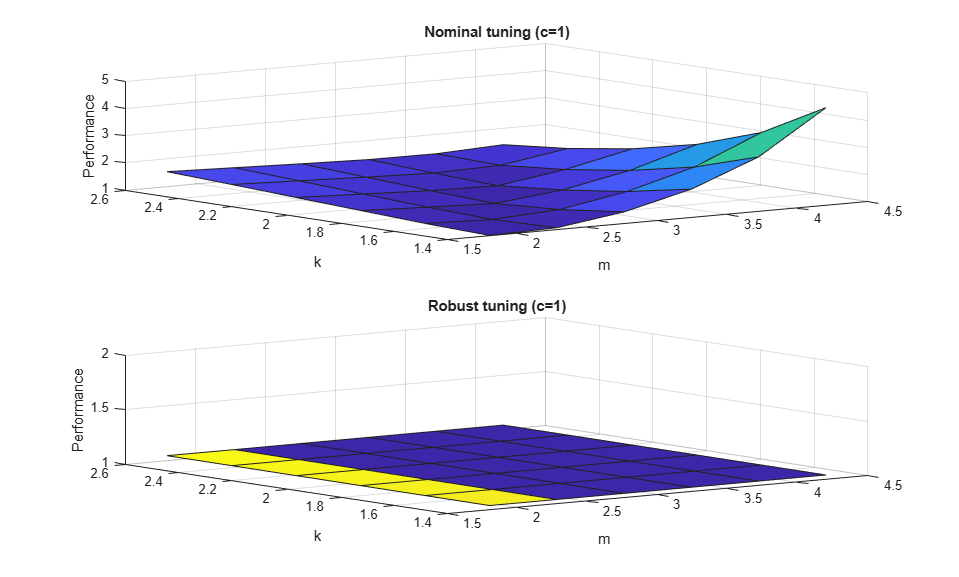Documentation

## Robust Tuning of Mass-Spring-Damper System

This example shows how to robustly tune a PID controller for an uncertain mass-spring-damper system modeled in Simulink.

### Simulink Model of Mass-Spring-Damper System

The mass-spring-damper depicted in Figure 1 is modeled by the second-order differential equation

``

whereis the force applied to the mass andis the horizontal position of the mass.Figure 1: Mass-Spring-Damper System.

This system is modeled in Simulink as follows:

```open_system('rct_mass_spring_damper') ```We can use a PID controller to generate the effortneeded to change the position. Tuning this PID controller is easy when the physical parametersare known exactly. However this is rarely the case in practice, due to a number of factors including imprecise measurements, manufacturing tolerances, changes in operating conditions, and wear and tear. This example shows how to take such uncertainty into account during tuning to maintain high performance within the range of expected values for.

### Uncertainty Modeling

The Simulink model uses the "most probable" or "nominal" values of:

``

Use the "uncertain real" (`ureal`) object to model the range of values that each parameter may take. Here the uncertainty is specified as a percentage deviation from the nominal value.

```um = ureal('m',3,'Percentage',40); uc = ureal('c',1,'Percentage',20); uk = ureal('k',2,'Percentage',30); ```

### Nominal Tuning

First tune the PID controller for the nominal parameter values. Here we use two simple design requirements:

• Positionshould track a step change with a 1 second response time

• Filter coefficientin PID controller should not exceed 100.

These requirements are expressed as tuning goals:

```Req1 = TuningGoal.Tracking('r','x',1); Req2 = TuningGoal.ControllerPoles('Controller',0,0,100); ```

Create an `slTuner` interface for tuning the "Controller" block in the Simulink model, and use `systune` to tune the PID gains and best meet the two requirements.

```ST0 = slTuner('rct_mass_spring_damper','Controller'); ST = systune(ST0,[Req1 Req2]); ```
```Final: Soft = 1.02, Hard = -Inf, Iterations = 44 ```

Use `getIOTransfer` to view the closed-loop step response.

```Tnom = getIOTransfer(ST,'r','x'); step(Tnom) ```The nominal response meets the response time requirement and looks good. But how robust is it to variations of?

### Robustness Analysis

To answer this question, use the "block substitution" feature of `slTuner` to create an uncertain closed-loop model of the mass-spring-damper system. Block substitution lets you specify the linearization of a particular block in a Simulink model. Here we use this to replace the crisp values ofby the uncertain values `um,uc,uk` defined above.

```blocksubs(1).Name = 'rct_mass_spring_damper/Mass'; blocksubs(1).Value = 1/um; blocksubs(2).Name = 'rct_mass_spring_damper/Damping'; blocksubs(2).Value = uc; blocksubs(3).Name = 'rct_mass_spring_damper/Spring Stiffness'; blocksubs(3).Value = uk; UST0 = slTuner('rct_mass_spring_damper','Controller',blocksubs); ```

To assess the robustness of the nominal tuning, apply the tuned PID gains to the (untuned) uncertain model `UST0` and simulate the "uncertain" closed-loop response.

```% Apply result of nominal tuning (ST) to uncertain closed-loop model UST0 setBlockValue(UST0,getBlockValue(ST)); Tnom = getIOTransfer(UST0,'r','x'); rng(0), step(Tnom,25), grid ```The `step` plot shows the closed-loop response with the nominally tuned PID for 20 randomly selected values ofin the specified uncertainty range. Observe the significant performance degradation for some parameter combinations, with poorly damped oscillations and a long settling time.

### Robust Tuning

To improve the robustness of the PID controller, re-tune it using the uncertain closed-loop model `UST0` rather than the nominal closed-loop model `ST0`. Due to the presence of `ureal` components in the model, `systune` automatically tries to maximize performance over the entire uncertainty range. This amounts to minimizing the worst-case value of the "soft" tuning goals `Req1` and `Req2`.

```UST0 = slTuner('rct_mass_spring_damper','Controller',blocksubs); UST = systune(UST0,[Req1 Req2]); ```
```Soft: [1.02,4.91], Hard: [-Inf,-Inf], Iterations = 44 Soft: [1.03,1.41], Hard: [-Inf,-Inf], Iterations = 31 Soft: [1.04,1.04], Hard: [-Inf,-Inf], Iterations = 21 Final: Soft = 1.04, Hard = -Inf, Iterations = 96 ```

The robust performance is only slightly worse than the nominal performance, but the same uncertain closed-loop simulation shows a significant improvement over the nominal design.

```Trob = getIOTransfer(UST,'r','x'); rng(0), step(Tnom,Trob,25), grid legend('Nominal tuning','Robust tuning') ```This is confirmed by plotting the worst-case gain fromtoas a function of frequency. Note the attenuated resonance near 1 rad/s.

```clf subplot(121), wcsigma(Tnom,{1e-2,1e2}), grid set(gca,'YLim',[-20 10]), title('Nominal tuning') subplot(122), wcsigma(Trob,{1e-2,1e2}), grid set(gca,'YLim',[-20 10]), title('Robust tuning'), legend('off') ```A comparison of the two PID controllers shows similar behaviors except for one key difference. The nominally tuned PID excessively relies on "cancelling" (notching out) the plant resonance, which is not a robust strategy in the presence of uncertainty on the resonance frequency.

```Cnom = getBlockValue(ST,'Controller'); Crob = getBlockValue(UST,'Controller'); clf, bode(Cnom,Crob), grid legend('Nominal tuning','Robust tuning') ```For further insight, plot the performance index (maximum value of the "soft" tuning goals `Req1,Req2`) as a function of the uncertain parametersfor the nominal damping. Use the "varying parameter" feature of `slTuner` to create an array of closed-loop models over a grid ofvalues covering their uncertainty ranges.

```% Specify a 6-by-6 grid of (m,k) values for linearization ms = linspace(um.Range(1),um.Range(2),6); ks = linspace(uk.Range(1),uk.Range(2),6); [ms,ks] = ndgrid(ms,ks); params(1).Name = 'm'; params(1).Value = ms; params(2).Name = 'k'; params(2).Value = ks; STP = slTuner('rct_mass_spring_damper','Controller',params); % Evaluate performance index over (m,k) grid for nominally tuned PID setBlockValue(STP,'Controller',Cnom) [~,F1] = evalGoal(Req1,STP); [~,F2] = evalGoal(Req2,STP); Fnom = max(F1,F2); % Evaluate performance index over (m,k) grid for robust PID setBlockValue(STP,'Controller',Crob) [~,F1] = evalGoal(Req1,STP); [~,F2] = evalGoal(Req2,STP); Frob = max(F1,F2); % Compare the two performance surfaces clf subplot(211), surf(ms,ks,Fnom) xlabel('m'), ylabel('k'), zlabel('Performance'), title('Nominal tuning (c=1)') subplot(212), surf(ms,ks,Frob), set(gca,'ZLim',[1 2]) xlabel('m'), ylabel('k'), zlabel('Performance'), title('Robust tuning (c=1)') ```This plot shows that the nominal tuning is very sensitive to changes in massor spring stiffness, while the robust tuning is essentially insensitive to these parameters. To complete the design, use `writeBlockValue` to apply the robust PID gains to the Simulink model and proceed with further validation in Simulink.

```writeBlockValue(UST) ```
##### SupportGet trial now